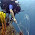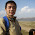## Wednesday, October 28, 2015

### Handy little snippet of R code for thinning occurrence data

I came up with this a few months back.  I was using the R package spThin, by Aiello-Lammens et al, but found that it didn't quite do what I wanted it to do.  The purpose of that package is to return the maximum number of records for a given thinning distance, which is obviously very valuable in situations where you (1) don't have a ton of data and (2) are concerned about spatial autocorrelation.

However, it wasn't quite what I needed for two reasons.  First, I didn't need to maximize the number of points given a specified thinning distance; I needed to grab a fixed number of points that did the best possible job of spanning the variation (spatial or otherwise) in my initial data set.  Second, the spThin algorithm, because it's trying to optimize sample size, can take a very long time to run for larger data sets.

Here's the algorithm I fudged together:

1. Pick a single random point from your input data set X and move it to your output set Y.

Then, while the number of points in Y is less than the number you want:
2. Calculate the distance between the points in X and the points in Y.
3. Pick the point from X that has the highest minimum distance to points in Y (i.e., is the furthest away from any of the points you've already decided to keep).

Lather, rinse, repeat.  Stop when you've got the number of points you want.

Just so you can get an idea of what's going on, here's some data on Leucadendron discolor from a project I'm working on with Haris Saslis-Lagoudakis:

That's a lot of points!  4,874, to be exact.  Now let's run it through thin.max and ask for 100 output points:

Lovely!  We've got a pretty good approximation of the spatial coverage of the initial data set, but with a lot fewer points.  What's extra-nice about this approach is that you can use it for pretty much any set of variables that you can use to calculate Euclidean distances.  That means you can thin data based on geographic distance, environmental distance, or a combination of both!  Just pass it the right column names, and it should work.  Of course since it's Euclidean distances you might run into some scaling issues if you try to use a combination of geographic and environmental variables, but you could probably fix that by rescaling axes before selecting points in some useful way.

Also, since it starts from a randomly chosen point, you will get somewhat different solutions each time you run it.  Could be useful for some sort of spatially-overdispersed jackknife if you want to do something like that for some reason.

There's no R package as such for this, but it will probably be folded into the R version of ENMTools when I get around to working on that again.  For now, you can get the code here:

https://gist.github.com/danlwarren/271288d5bab45d2da549

#### 7 comments:

1.Hi Dan, I was just working on a similar problem. Though I wanted to randomly sample a set of point, enforcing a minimum distance between them. And like you, I didn't care about maximizing the number of points, I just wanted it to be random. I deviate somewhat from your method, maybe because we had different motivations for doing this. Basically I did:

1) randomly chose one point from the original, and move it to a new set (like you)
2) calculate the distance between this point, and all of the rest left in the original (like you)
3) delete from the original those points that fall within the minimum distance of the point moved to the new set
4) repeat until there is nothing left in the original set

2.Funny enough, I had thought of doing essentially the same thing when I was originally writing this bit of code. You could get the same results from this code just by making the stop condition a minimum distance instead of a fixed number of points.

3.May be try this method in the following paper.
http://www.sciencedirect.com/science/article/pii/0377042796000350
Which one is better?

4.Definitely seems applicable, but I can't immediately see what advantages it might have over this method. Do you have an idea?

5.Hi, Dan
I am not sure which is better. My guess is that the results may be similar. But I see the computation time of yours and their thinning algorithm are both O(N^3) algorithmic complexity, but theirs should be faster than yours.
https://en.wikipedia.org/wiki/Computational_complexity_theory
Could you tell me how much time does it take for your case? It looks like you also tried spThin and it is slower?
My application is addressing millions of data points. So I wrote a program much faster but not stricter:
Spatial points are overlayed with spatial grids with a specified cell size and then get a subset from each grid with a specified number at most. If one grid has less points than the specified number, all the points are taken. If one grid has more points than the specified number, only this number of points are taken by sample. This function can be used when there are too much point observations to be handled, especially for spatially clustered observations.

I put it into the GSIF package.
http://gsif.r-forge.r-project.org/sample.grid.html

6.Yeah that definitely seems like it's going to be way faster when you've got a whole bunch of points - even though this method is miles faster than spThin, it still gets quite time-consuming when you get more than a few tens of thousands of points. One thing that this method can do that I don't think sample.grid can do (unless I misunderstand) is thin points based on distance in any number of dimensions, not just spatial. You could use this algorithm (and the earlier one you posted, I think) to thin points in environment space as well as (or instead of) geographic space.

Anyway, I don't have time to muck about with it much at the moment, I have a class to prepare. Cheers!

7.Hi, Dan. Thank you for your reply
sample.grid can also extent to n dimension by splitting the space into cube or hyper-cube if necessary. The disadvantage are that the spatial auto-correlation within a grid is not considered, and one can not determine the number of the output samples.
But it is possible to use your method or similar in each grid instead of the random sampling in sample.grid. In this way, we can find a balance between the spatial autocorrelation and the computing time.
Anyway, this is also not my focus right now. Just keep in mind. Cheers!